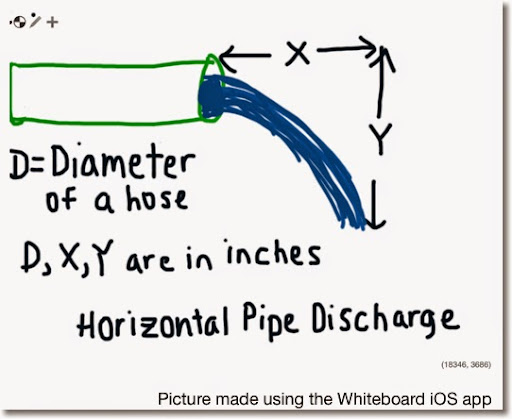## Sunday, May 25, 2014

### PCalc and HP 50g: Horizontal Pipe DischargePCalc and HP 50g: Horizontal Pipe Discharge

Variables:
X, Y, and D (diameter of the pipe) are in inches. Flow (Q) is calculated in GPM (gallons per minute).

Q ≈ .25974 * π * D^2/4 * X * √( g /(2 * Y))

Where
g = 9.80665 m/s^2 ≈ 386.08858 in/s^2
1 in^3/s ≈ .25974 gal/min

Source:

Gary P. Markey. Lecture 14: Flow Measurement in Pipes. BIE 5300/6300 Lectures
Utah State University - Open Courseware

http://ocw.usu.edu/Biological_and_Irrigation_Engineering/Irrigation___Conveyance_Control_Systems/L14_FlowMeasurementInPipes.pdf

PCalc: Horiz. Pipe Discharge (GPM)

Store the following values before proceeding:
D in M1
X in M2
Y in M3

Decimal Mode
Set X To M1
X To Power of 2
Multiply X By M2
Multiply X By Pi
Divide X By 4
Set R0 To 386.08858
Divide R0 By 2
Divide R0 By M3
R0 To Power of 0.5
Multiply X By R0
Multiply X By .25974

HP 50g: HPD

SQ: x^2

<< "Hose Diameter (in)" PROMPT
"X (in)" PROMPT
"Y (in)" PROMPT
→ D X Y
<< π D SQ * X * 4 / →NUM
386.08858 2 Y * / √ *
.25974 * "Flow (GPM)" →TAG >> >>

Example:
D = 2.5 in
X = 8 in
Y = 4 in

Result:
Flow ≈ 70.85936 Gallons per Minute

- Eddie -

### Casio fx-CG50: Sparse Matrix Builder

Casio fx-CG50: Sparse Matrix Builder Introduction The programs can create a sparse matrix, a matrix where most of the entries have zero valu...